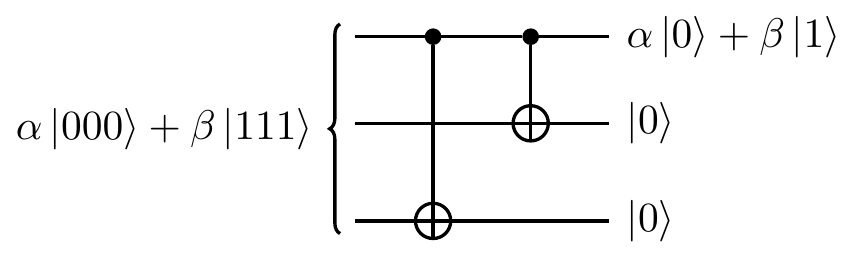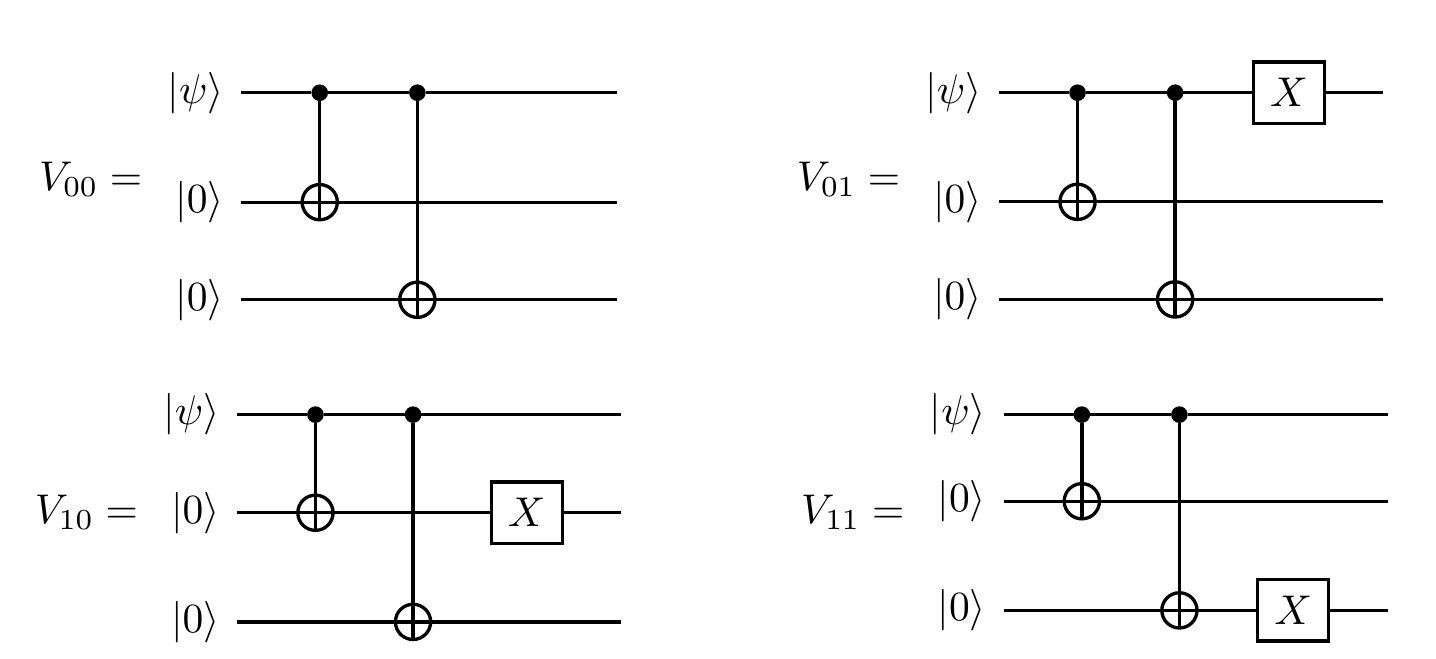## 13.5 Repetition codes

In order to give a sense of how quantum error correction actually works, let us begin with a classical example of a repetition code.

Suppose that Alice is communicating to Bob over a channel which, with some probability p, flips each (classical) bit that she transmits.258 Even if Bob knows that these potential flips are happening, he cannot always compensate for the errors introduced. It could be the case that he receives the binary message, converts it into alphabetic characters, and reads “Hello Bpb”. Then he would likely think to himself that Alice had intended to send “Hello Bob”, and no problems would arise. But if the transmitted message instead read “Hellp Bob”, then he might be less sure — maybe Alice had just meant to send “Hello Bob”, but maybe she had instead meant to send “Help Bob”. Of course, if the probability p is high enough, then the actual messages that Bob receives could be more along the lines of “ytlF#kb2F”, and at this point both he and Alice decide that they need some way to accommodate for the error-inducing communications channel.

One thing that they might try is to encode each bit into three bits: \begin{aligned} 0 &\mapsto 000 \\1 &\mapsto 111. \end{aligned} That is, each time Alice wants to send a single logical 0, she instead sends three physical bits, all in state 0; each time she wants to send a single logical 1, she instead sends three physical bits, all in state 1. Bob decodes the bit value by a “majority vote” of the three bits. If only one error occurs in a given “block” of three bits, then this error correction procedure is foolproof. In general, the net probability of error in a received block is just the likelihood that either two or three errors occur, which is 3p^2(1-p) + p^3. If p<\frac{1}{2}, then this probability is indeed less than p, and so the three-bit code improves the reliability of the information transfer.

The quantum case, however, is more complicated, because we have to worry about both bit-flip and phase-flip errors.

But let’s start simply: how can we protect a qubit against a single bit-flip error X? It turns out that we can actually rely on the same triple-repetition code, but we just need to use the language of quantum operations.

Given some qubit in an unknown pure state \alpha|0\rangle + \beta|1\rangle, we encode it into three qubits by using \texttt{c-NOT} gates:Suppose that the second qubit is flipped by our noisy channel, so that the encoded state becomes \alpha|010\rangle + \beta|101\rangle. Decoding this requires some care: if we measure the three qubits directly it would destroy the superposition of states that we are working so hard to protect. So instead we introduce another two additional qubits, which we refer to as ancilla bits, both in state |0\rangle, and apply the following encoding network:We measure the two ancilla bits (in the standard basis), and the result of the measurement, known as the error syndrome, tells us how to reset the three qubits of the code, as follows.

The first ancilla compares qubits one and two (counting from the top), and the second ancilla compares qubits two and three: if the ancilla is measured and found to be the |0\rangle state, then it means that the corresponding qubits were in the same state; if it is found in the |1\rangle state, then the corresponding qubits were in different states. Hence, the four possible error syndromes — |00\rangle, |01\rangle, |10\rangle, and |11\rangle — each indicate a different possibility: no errors, or an error in the third, first, or second qubits, respectively. In our example we would measure |11\rangle, revealing that the first two qubits were in different states, as were the last two qubits, and so the second qubit must have been flipped. Knowing the error, we can go back and fix it, simply by applying X to the second qubit. The net result is the state \alpha|000\rangle + \beta|111\rangle, which is then turned into (\alpha|0\rangle + \beta|1\rangle)|0\rangle|0\rangle by running the mirror image of the encoding network.

This three-qubit code259 that we have just demonstrated is sufficient to protect a qubit against single bit-flips, but not phase-flips. But if we know that we only have to worry about phase-flips, not bit-flips, then this is actually enough! Recall that HZH=X, and so it is enough to sandwich the decoherence area in between the Hadamard gates: they will turn phase-flips into bit-flips, and we have just seen how to protect our qubits against X-errors. Then the encoded state \alpha|0\rangle+\beta|1\rangle now reads \alpha|+++\rangle+\beta|---\rangle, where |\pm\rangle=|0\rangle\pm|1\rangle. That is, to deal with phase-flips instead of bit-flips, we simply use the \{|+\rangle,|-\rangle\} basis instead of the \{|0\rangle,|1\rangle\} basis.

Finally then, we can consider how to deal with both types of errors at once. First we encode the qubit using the phase-flip code, and then we encode each of the resulting three qubits of the code using the bit-flip code. This gives an error correction scheme that allows us to protect against both types of error by encoding a single logical qubit across nine physical qubits, protecting against a single quantum error on any of the nine qubits.

If we want to preserve a quantum state for a long time without doing any computations, or if we want to send it through a noisy communications channel, we can just encode the state using a quantum code and decode it when we are done. Computation on encoded states using noisy gates, however, requires a few more tricks…

1. This scenario could arise from the channel being noisy, damaged, or even maliciously modified by Eve.↩︎

2. The code is called a “three-qubit code” because this is how many we need to encode it, but to decode it we actually need five qubits, since we need the ancillas.↩︎